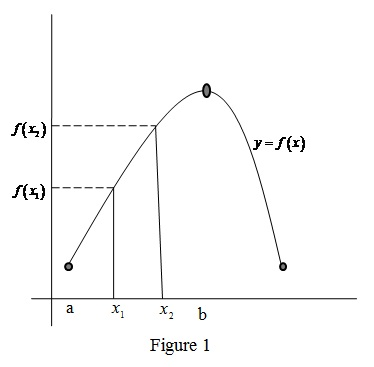# An increasing function.### Single Variable Calculus: Concepts...

4th Edition
James Stewart
Publisher: Cengage Learning
ISBN: 9781337687805### Single Variable Calculus: Concepts...

4th Edition
James Stewart
Publisher: Cengage Learning
ISBN: 9781337687805

#### Solutions

Chapter 1, Problem 4RCC
To determine

## To define: An increasing function.

Expert Solution

### Explanation of Solution

Consider a function y=f(x).

Let two points x1 and x2 such that x1<x2 lie on the closed interval [a, b].

The function y=f(x) is said be an increasing function on the given interval [a, b] if f(x1)<f(x2) whenx1<x2.

Graph:

The behavior of an increasing function is shown below in Figure 1.From Figure 1, it is observed that the curve y=f(x) is increasing on the interval [a, b].

### Have a homework question?

Subscribe to bartleby learn! Ask subject matter experts 30 homework questions each month. Plus, you’ll have access to millions of step-by-step textbook answers!